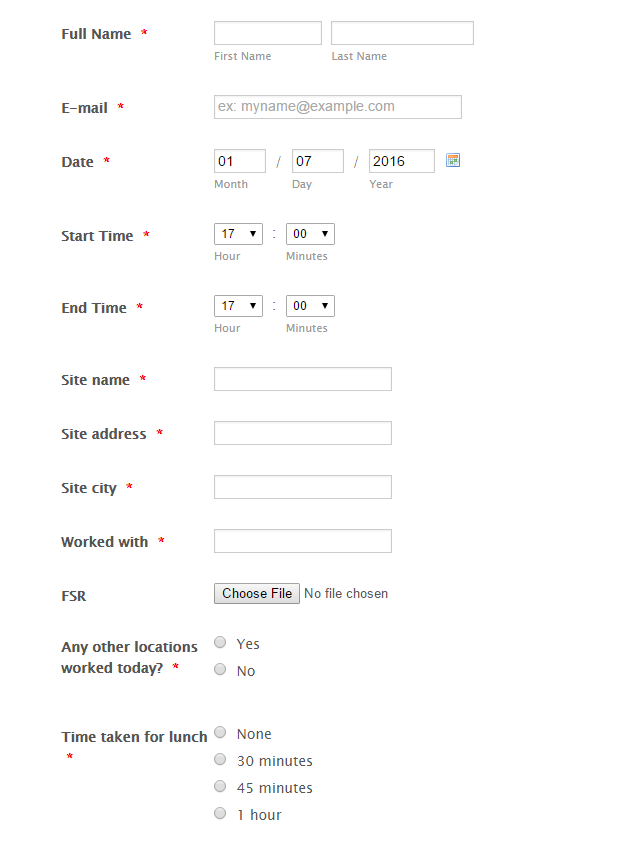Can field auto calculate?

•TracyMoralee
Asked on January 07, 2016 at 04:57 PM

I am creating a form to use for employees to enter their time worked like a timesheet. Is there a way to have the form auto calculate the total number of hours worked? I have a field where they add their start time, a field to enter their end time and then another field where they select whether they took a 30 min, 45 minute or 1 hour lunch or none.

Thank you!

PS I am using the 24 hour clock•EltonCris
Answered on January 07, 2016 at 10:56 PM

Yes, form calculation is possible. Here's a guide for that: http://www.jotform.com/help/259-How-to-perform-calculation-in-the-form

If you need further help, let us know.

•TracyMoralee
Answered on January 08, 2016 at 01:28 AM

Thank you! I will try that!

•EltonCris
Answered on January 08, 2016 at 02:37 AM

Great!

Feel free to let us know if you need further assistance.

Regards!

•Jeanette
Answered on January 11, 2016 at 04:13 PM

Hello,

I have added the equation using a form calculation widget and it is not calculating. I am not sure what I am doing wrong.

Thank you!

•Chriistian
Answered on January 11, 2016 at 11:24 PM

Hi,

It looks like there's a problem with the formula. Once the user selects the 30 minute or 45 minute option, the formula subtracts it by either 30 or 45.It also looks like the calculation values for the options are empty. YYou can check the calculation value by clicking on the gear icon then select Edit Properties.Then to fix the formula, add these values to the calculation field:Do inform us if you need further assistance,
Regards.